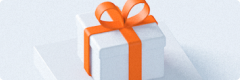API接口，开发服务，免费咨询服务

# 在canvas中模拟光照效果 #2

## 平行光源

``````/**
* 点乘运算
* @param {Array<number>} v1 向量v1
* @param {Array<number>} v2 向量v2
* @return {number} 点乘结果
*/
function dot( v1, v2 ) {
return v1[ 0 ] * v2[ 0 ] + v1[ 1 ] * v2[ 1 ] + v1[ 2 ] * v2[ 2 ];
}
``````

``````/**
* 将向量转为单位向量
* @param {Array<number>} v
* @return {Array<number>} 单位向量
*/
function normalize( v ) { /* ... */ }

/**
* 两向量相减
* @param {Array<number>} v1
* @param {Array<number>} v2
* @return {Array<number>}
*/
function sub( v1, v2 ) { /* ... */ }

/**
* 计算一个向量的反方向向量
* @param {Array<number>} v
* @return {Array<number>}
*/
function negate( v ) { /* ... */ }
``````

``````var plane = {
center: [ 250, 250, 0 ],    // 平面中心点坐标
width: 500,                 // 宽
height: 500,                // 高
normal: [ 0, 0, 1 ],        // 朝向，即法向量
color: { r: 255, g: 0, b: 0 }   // 颜色为红色
}``````

``````var directionalLight = {
direction: [ 0, 0, -1 ],        // 从屏幕外垂直照向屏幕
color: { r: 255, g: 255, b: 255 }   // 颜色为纯白色
}``````

``````// ...
var reverseLightDirection = negate( directionalLight.direction );   // 计算平行光的反方向向量
var intensity = dot( reverseLightDirection, plane.normal );         // 计算两向量点乘

// 计算有光照时的颜色
var color = {
r: intensity * plane.color.r + intensity * directionalLight.r,
g: intensity * plane.color.g + intensity * directionalLight.g,
b: intensity * plane.color.b + intensity * directionalLight.g,
}

var canvas = document.getElementById( 'canvas' );
var ctx = canvas.getElementById( '2d' );
ctx.rect( plane.center[ 0 ], plane.center[ 1 ], plane.width, plane.height );
ctx.fillStyle = 'rgb(' + color.r + ',' + color.g + ',' + color.b ')';
ctx.fill();``````

### 点光源

``````var pointLight = {
position: [ 250, 250, 100 ],    // 光源位于平面中心上方100处
color: { r: 255, g: 255, b: 255 }   // 颜色为纯白色
}``````

``````// ...
var imageData = ctx.createImageData( 500, 500 );    // 创建一个ImageData，用来保存像素数据

for ( var x = 0; x < imageData.width; x++ ) {
for ( var y = 0; y < imageData.height; y++ ) {
var index = y * imageData.width + x;        // 当前计算的像素点的索引

var point = [ x, y, 0 ];
var normal = [ 0, 0, 1 ];

var reverseLightDirection = normalize( sub( pointLight.position, point ) );  // 光线方向的反方向向量

var light = dot( reverseLightDirection, normal );

imageData.data[ index * 4 ] = pointLight.color.r * intensity + plane.color.r * intensity;
imageData.data[ index * 4 + 1 ] = pointLight.color.g * intensity + plane.color.g * intensity;
imageData.data[ index * 4 + 2 ] = pointLight.color.b * intensity + plane.color.b * intensity;
imageData.data[ index * 4 + 3 ] = 255;
}
}

ctx.putImageData( imageData, 100, 100 );``````

WebGL渲染的光照效果

## 关于我的博客

#### API资讯

API接口，开发服务，免费咨询服务

## 平行光源

``````/**
* 点乘运算
* @param {Array<number>} v1 向量v1
* @param {Array<number>} v2 向量v2
* @return {number} 点乘结果
*/
function dot( v1, v2 ) {
return v1[ 0 ] * v2[ 0 ] + v1[ 1 ] * v2[ 1 ] + v1[ 2 ] * v2[ 2 ];
}
``````

``````/**
* 将向量转为单位向量
* @param {Array<number>} v
* @return {Array<number>} 单位向量
*/
function normalize( v ) { /* ... */ }

/**
* 两向量相减
* @param {Array<number>} v1
* @param {Array<number>} v2
* @return {Array<number>}
*/
function sub( v1, v2 ) { /* ... */ }

/**
* 计算一个向量的反方向向量
* @param {Array<number>} v
* @return {Array<number>}
*/
function negate( v ) { /* ... */ }
``````

``````var plane = {
center: [ 250, 250, 0 ],    // 平面中心点坐标
width: 500,                 // 宽
height: 500,                // 高
normal: [ 0, 0, 1 ],        // 朝向，即法向量
color: { r: 255, g: 0, b: 0 }   // 颜色为红色
}``````

``````var directionalLight = {
direction: [ 0, 0, -1 ],        // 从屏幕外垂直照向屏幕
color: { r: 255, g: 255, b: 255 }   // 颜色为纯白色
}``````

``````// ...
var reverseLightDirection = negate( directionalLight.direction );   // 计算平行光的反方向向量
var intensity = dot( reverseLightDirection, plane.normal );         // 计算两向量点乘

// 计算有光照时的颜色
var color = {
r: intensity * plane.color.r + intensity * directionalLight.r,
g: intensity * plane.color.g + intensity * directionalLight.g,
b: intensity * plane.color.b + intensity * directionalLight.g,
}

var canvas = document.getElementById( 'canvas' );
var ctx = canvas.getElementById( '2d' );
ctx.rect( plane.center[ 0 ], plane.center[ 1 ], plane.width, plane.height );
ctx.fillStyle = 'rgb(' + color.r + ',' + color.g + ',' + color.b ')';
ctx.fill();``````

### 点光源

``````var pointLight = {
position: [ 250, 250, 100 ],    // 光源位于平面中心上方100处
color: { r: 255, g: 255, b: 255 }   // 颜色为纯白色
}``````

``````// ...
var imageData = ctx.createImageData( 500, 500 );    // 创建一个ImageData，用来保存像素数据

for ( var x = 0; x < imageData.width; x++ ) {
for ( var y = 0; y < imageData.height; y++ ) {
var index = y * imageData.width + x;        // 当前计算的像素点的索引

var point = [ x, y, 0 ];
var normal = [ 0, 0, 1 ];

var reverseLightDirection = normalize( sub( pointLight.position, point ) );  // 光线方向的反方向向量

var light = dot( reverseLightDirection, normal );

imageData.data[ index * 4 ] = pointLight.color.r * intensity + plane.color.r * intensity;
imageData.data[ index * 4 + 1 ] = pointLight.color.g * intensity + plane.color.g * intensity;
imageData.data[ index * 4 + 2 ] = pointLight.color.b * intensity + plane.color.b * intensity;
imageData.data[ index * 4 + 3 ] = 255;
}
}

ctx.putImageData( imageData, 100, 100 );``````

WebGL渲染的光照效果

## 关于我的博客

• 短信API服务
• 银行卡四元素校验
• 身份证实名认证
• 手机状态查询
• 三网手机实名制认证（简版）
• 身份证OCR识别
• 风险信息查询
• 企业工商信息

• 短信API服务
• 银行卡四元素校验
• 身份证实名认证
• 手机状态查询
• 三网手机实名制认证（简版）
• 身份证OCR识别
• 风险信息查询
• 企业工商信息
• 确定×

×立即领取
× 企业用户认证，

Data Drives The Future